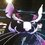# Algebra Mania!

Hello, I propose this problem to the Brilliant community. Hope you enjoy it! This problem was one of the questions in Olympiads.

What is the remainder obtained of the long division $$\large \dfrac{x^{81}+x^{49}+x^{25}+x^{9}+x}{x^3-x}$$?Note by Puneet Pinku
2 years, 8 months ago

MarkdownAppears as
*italics* or _italics_ italics
**bold** or __bold__ bold
- bulleted- list
• bulleted
• list
1. numbered2. list
1. numbered
2. list
Note: you must add a full line of space before and after lists for them to show up correctly
paragraph 1paragraph 2

paragraph 1

paragraph 2

[example link](https://brilliant.org)example link
> This is a quote
This is a quote
    # I indented these lines
# 4 spaces, and now they show
# up as a code block.

print "hello world"
# I indented these lines
# 4 spaces, and now they show
# up as a code block.

print "hello world"
MathAppears as
Remember to wrap math in $$...$$ or $...$ to ensure proper formatting.
2 \times 3 $$2 \times 3$$
2^{34} $$2^{34}$$
a_{i-1} $$a_{i-1}$$
\frac{2}{3} $$\frac{2}{3}$$
\sqrt{2} $$\sqrt{2}$$
\sum_{i=1}^3 $$\sum_{i=1}^3$$
\sin \theta $$\sin \theta$$
\boxed{123} $$\boxed{123}$$

## Comments

Sort by:

Top Newest

Can you just point out the mistake in my solution:

Let the remainder be r(x)=(Ax^2+Bx+C).

let P(x) be the polynomial on the numerator.

P(x)=(x^3-x)g(x)+r(x)

setting x=0,

P(0)=r(0)=C

or,C=0...................(1)

setting x=1,

P(1)=r(1)=A+B

or,A+B=5................(2)

setting x=-1,

P(-1)=r(-1)=A-B

or,A-B=-5..................(3)

Solving (1),(2),and (3), we get A=C=0,and B=5.

So, remainder=5x (ans)

- 2 years, 3 months ago

Log in to reply

The problem is, that x cannot be 0. That would make the denominator of the fraction zero (division by zero).

- 2 years, 3 months ago

Log in to reply

We see that after dividing by $$x$$, we have the expression

$$\frac{x^{80}+x^{48}+x^{24}+x^8+1}{(x+1)(x-1)}$$

Now consider

$$\frac{x^{80}+x^{48}+x^{24}+x^8+1}{(x+1)(x-1)}-\frac{5}{(x+1)(x-1)}$$

$$=\frac{x^{80}+x^{48}+x^{24}+x^8-4}{(x+1)(x-1)}$$

We see that $$x-1$$ and $$x+1$$ are factors of $$x^{80}+x^{48}+x^{24}+x^8-4$$ by the factor theorem as 1 and -1 are roots of this polynomial. Hence we can write

$$\frac{x^{80}+x^{48}+x^{24}+x^8-4}{(x+1)(x-1)}=\frac{x^{80}+x^{48}+x^{24}+x^8+1}{(x+1)(x-1)}-\frac{5}{(x+1)(x-1)}=p(x)+\frac{0}{(x+1)(x-1)}$$

For some polynomial $$p(x)$$

Therefore

$$\frac{x^{80}+x^{48}+x^{24}+x^8+1}{(x+1)(x-1)}=p(x)+\frac{5}{(x+1)(x-1)}$$.

- 2 years, 8 months ago

Log in to reply

One way to solve this is to simplify by the common factor x first, and then to use algebraic long division in a faster way ( " +...+ " after recognising the repetitive parts):

$$\frac {x^{80}+x^{48}+x^{24}+x^8+1}{x^2 - 1} = x^{78}+x^{76}+...+x^{48}+2x^{46}+2x^{44}+...+2x^{24}+3x^{22}+3x^{20}+...+3x^8+4x^6+4x^4+4x^2+4+ \frac { \boxed {5} }{x^2-1}$$

- 2 years, 8 months ago

Log in to reply

How to find that 1 will be the coefficient for this much time or 4 will be there for only few numbers and lastly 5 will come... I mean can you explain the pattern a bit more clearly...

- 2 years, 8 months ago

Log in to reply

For the algebraic (or polynomial) long division method in general, you can find many notes, videos etc. on the Internet (e.g. https://brilliant.org/wiki/polynomial-division/ or https://revisionmaths.com/advanced-level-maths-revision/pure-maths/algebra/algebraic-long-division ).

Just follow the method in the case of this division and you will see. (The coefficient increases at some points, because you will have the same powers of x from your remainder (at the previous step) and you also have an original term there (e.g. $$x^{48 }+ x^{48} = 2x^{46}$$

- 2 years, 8 months ago

Log in to reply

Did you perform the whole long division or somehow you analyzed and figured out the coefficients??? I recently found a new method to solve it..... I will be posting it as question.....

- 2 years, 8 months ago

Log in to reply

I started the whole, but jumped to the key points (where the remainders "got company" from the original polynomial) after recognising the pattern. With further analysis, the process can be shortened even further.

- 2 years, 8 months ago

Log in to reply

×

Problem Loading...

Note Loading...

Set Loading...